# efficiency

Since R2021a

## Syntax

``E = efficiency(antenna,frequency)``
``efficiency(antenna,frequency)``

## Description

example

````E = efficiency(antenna,frequency)` returns the radiation efficiency of the antenna over the specified frequency.```

example

````efficiency(antenna,frequency)` plots the radiation efficiency of the antenna over the specified frequency.```

## Examples

collapse all

Create a top-hat monopole antenna.

```m = metal('Lead'); ant = monopoleTopHat('Conductor',m); show(ant)```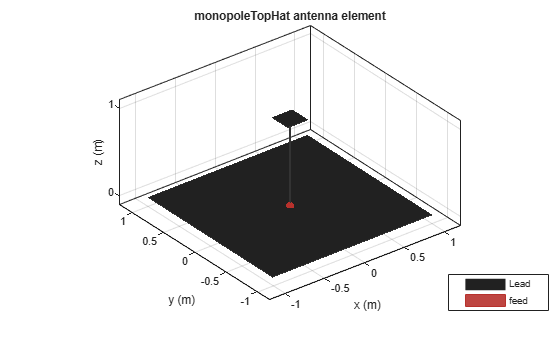Plot the radiation efficiency of the antenna over a frequency range of 40-100 MHz.

`efficiency(ant,linspace(40e6,100e6,41))`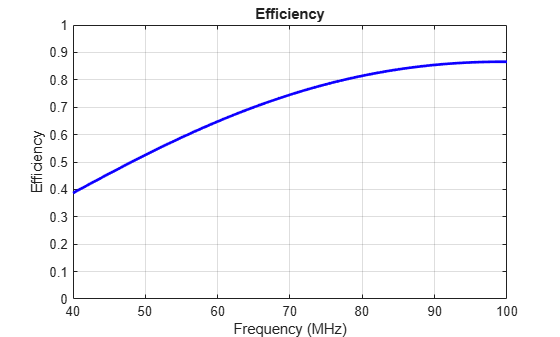Create and visualize patch microstrip antennas with PEC, copper, silver, and aluminium metal patches.

```ant_patchPEC = patchMicrostrip('Substrate',dielectric('Air'),'Conductor',metal('PEC')); ant_patchCopper = patchMicrostrip('Substrate',dielectric('FR4'),'Conductor',metal('Copper')); ant_patchSilver = patchMicrostrip('Substrate',dielectric('FR4'),'Conductor',metal('Silver')); ant_patchAluminium = patchMicrostrip('Substrate',dielectric('FR4'),'Conductor',metal('Aluminium'));```

Compare the radiation efficiency of these antennas at the frequency of 1 GHz.

`Eff_patchPEC = efficiency(ant_patchPEC,1e09)`
```Eff_patchPEC = 1 ```
`Eff_patchCopper = efficiency(ant_patchCopper,1e09)`
```Eff_patchCopper = 0.4918 ```
`Eff_patchSilver = efficiency(ant_patchSilver,1e09)`
```Eff_patchSilver = 0.4344 ```
`Eff_patchAluminium = efficiency(ant_patchAluminium,1e09)`
```Eff_patchAluminium = 0.4899 ```

## Input Arguments

collapse all

Input antenna, specified as an `antenna` object. The antenna object that you specify should be a single-feed structure.

Frequency range to calculate the radiation efficiency, specified as a scalar in hertz or a vector with each element unit in Hz.

Example: 50e6:1e6:100e6

Data Types: `double`

## Output Arguments

collapse all

Radiation efficiency of the antenna, returned as a scalar or a vector with values in the range [0,1].

collapse all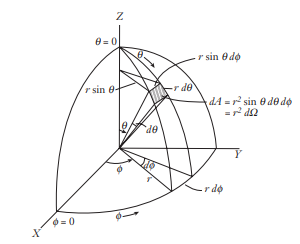The time-average power density radiated by an antenna is: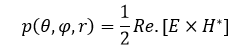where, r = radius of the sphere,

θ, φ = spherical coordinate angles,

E, H = electric and magnetic field intensity respectively.

The total power radiated by antenna is: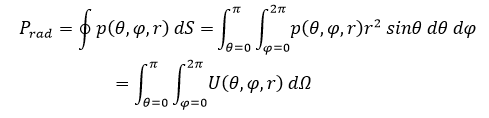where, U = radiation intensity (W/ unit solid angle),

dΩ = element of solid angle.

The radiation efficiency is given as: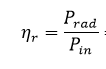where, Pin = total input power.

## Version History

Introduced in R2021a